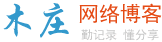# 如何在canvas里面基于随机点绘制一个多边形123456789101112131415161718192021222324 `let balls = [];``let ballNum = 6;``let firstBall = null;``while(ballNum--) {``  ``let ball = new Ball(20, parseColor(Math.random() * 0xffffff))``  ``ball.x = Math.random() * width;``  ``ball.y = Math.random() * height;``  ``balls.push(ball)` `  ``if (!firstBall) {``    ``firstBall = ball``    ``ball.angle = 0``  ``} else {``    ``const dx = ball.x - firstBall.x,``          ``dy = ball.y - firstBall.y;` `    ``ball.angle = Math.atan2(dy, dx);``  ``}``}` `// 尝试让球连线是一个正多边形``balls = balls.sort((ballA, ballB) => {``  ``return ballA.angle - ballB.angle``})`X轴两极点分割

 1234567891011121314151617181920212223242526272829303132333435363738394041424344454647484950515253545556575859606162636465666768697071727374757677787980 `let balls = [];``let tempBalls = [];``let ballNum = 6;``let isDragingBall = false;` `while(ballNum--) {``  ``let ball = new Ball(10, parseColor(Math.random() * 0xffffff))``  ``ball.x = Math.random() * width;``  ``ball.y = Math.random() * height;``  ``tempBalls.push(ball)``}` `// 让点按X轴升序排序``tempBalls = tempBalls.sort((ballA, ballB) => {``  ``return ballA.x - ballB.x``})` `// 找X轴左右极点``let firstBall = tempBalls,``    ``lastBall = tempBalls[tempBalls.length -1];``let smallXBalls = tempBalls.filter(ball => ball.x === firstBall.x),``    ``bigXBalls = tempBalls.filter(ball => ball.x === lastBall.x)` `// 处理左右极点有多个的情况``if (smallXBalls.length > 1) {``  ``smallXBalls.sort((ballA, ballB) => {``    ``return ballB.y - ballA.y``  ``})``}``if (bigXBalls.length > 1) {``  ``bigXBalls.sort((ballA, ballB) => {``    ``return ballB.y - ballA.y``  ``})``}` `firstBall = smallXBalls``lastBall = bigXBalls` `// 获得极点连线的角度``let splitLineAngle = Math.atan2(lastBall.y - firstBall.y, lastBall.x - firstBall.x);``let upperBalls = [],``    ``lowerBalls = [];` `// 所有其他点跟firstBall计算角度``// 大于splitLineAngle的都是下链``// 其他是上链``tempBalls.forEach(ball => {``  ``if (ball === firstBall || ball === lastBall) {``    ``return false``  ``}``  ``let angle = Math.atan2(ball.y - firstBall.y, ball.x - firstBall.x);``  ``if (angle > splitLineAngle) {``    ``lowerBalls.push(ball)``  ``} else {``    ``upperBalls.push(ball)``  ``}``})` `// 处理X轴相同情况的排序``lowerBalls = lowerBalls.sort((ballA, ballB) => {``  ``if (ballA.x !== ballB.x) {``    ``return ballA.x - ballB.x``  ``}``  ``return ballB.y - ballA.y``})` `upperBalls = upperBalls.sort((ballA, ballB) => {``  ``if (ballA.x !== ballB.x) {``    ``return ballB.x - ballA.x``  ``}``  ``return ballB.y - ballB.x``})` `// 逆时针连接所有的点``balls = [firstBall].concat(lowerBalls, [lastBall], upperBalls)` `balls = balls.map((ball, i) => {``  ``ball.text = i + 1;``  ``return ball``})`HTML5的Canvas实现绘制曲线的方法### 评论

• 欢迎访问木庄网络博客
• 可复制：代码框内的文字。
• 方法：Ctrl+C。